Convert CB Transceiver to 50MHz

An old CB transceiver is converted to the 50MHz amateur band using an Arduino Pro Mini, DDS, PLL and OLED display.

ExpertWork in progressOver 1 day24,901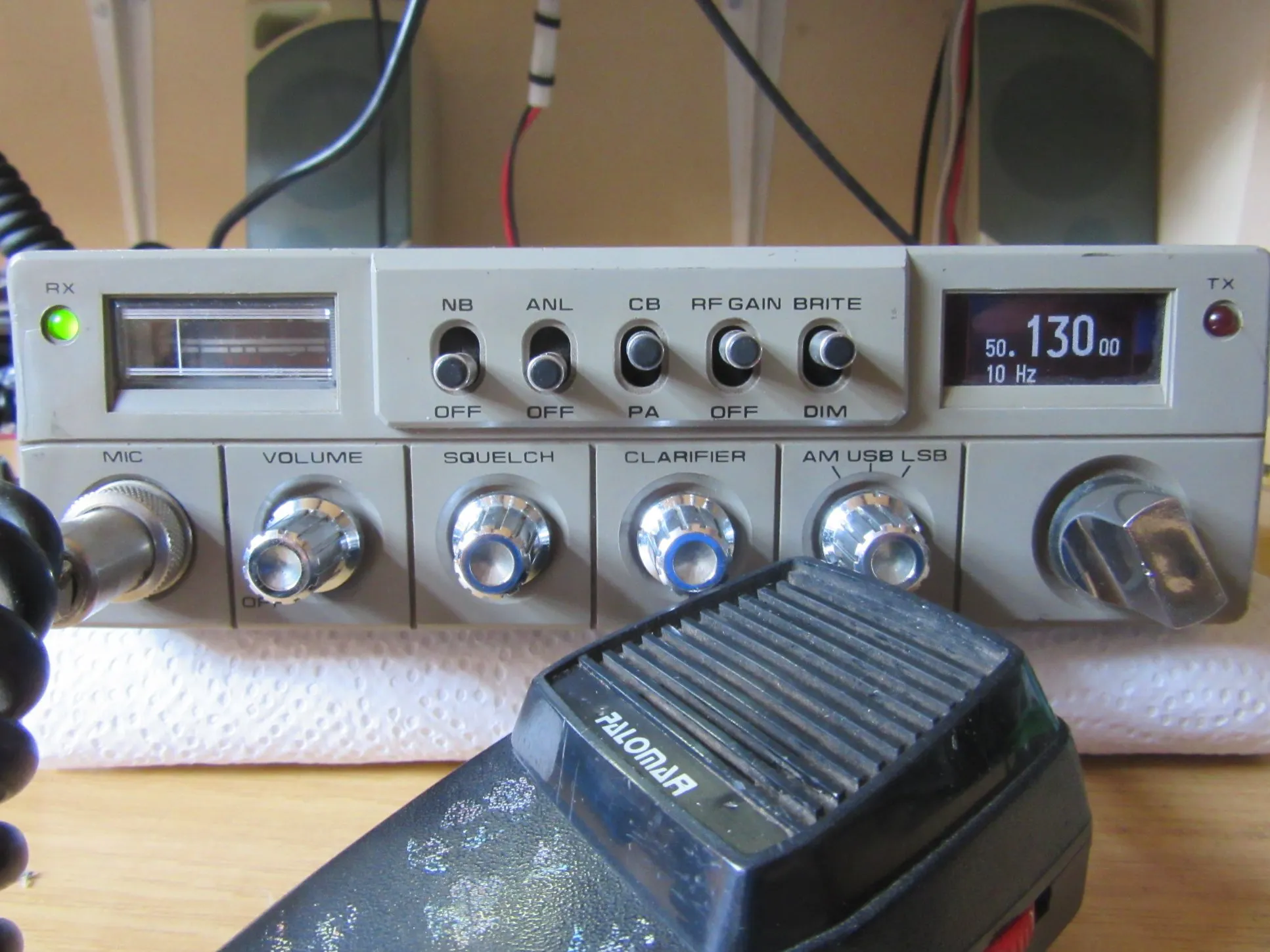Things used in this project

Hardware components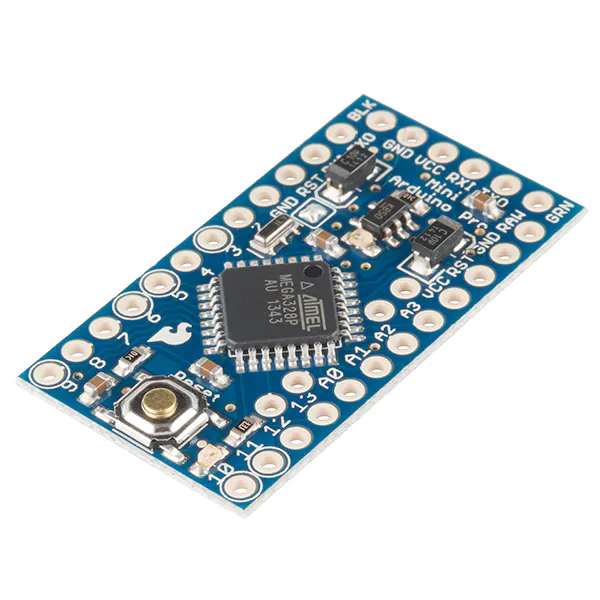SparkFun Arduino Pro Mini 328 - 5V/16MHz
×1
×1
 NXP MC145170 PLL
×1
 Oled 128x64 0.96" SPI
×1
 Mitsubishi PowerFET RD16HHF1
×1

Software apps and online services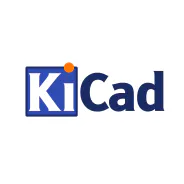KolourPaintArduino IDE

Hand tools and fabrication machinesSoldering iron (generic)
 Spectrumanalyser HP141T

Schematics

Schematic diagram of the additional PCB

PCB with the Arduino , PLL and DDS

Schematic diagram of modified CB transceiver

In red are the modifications of the transceiver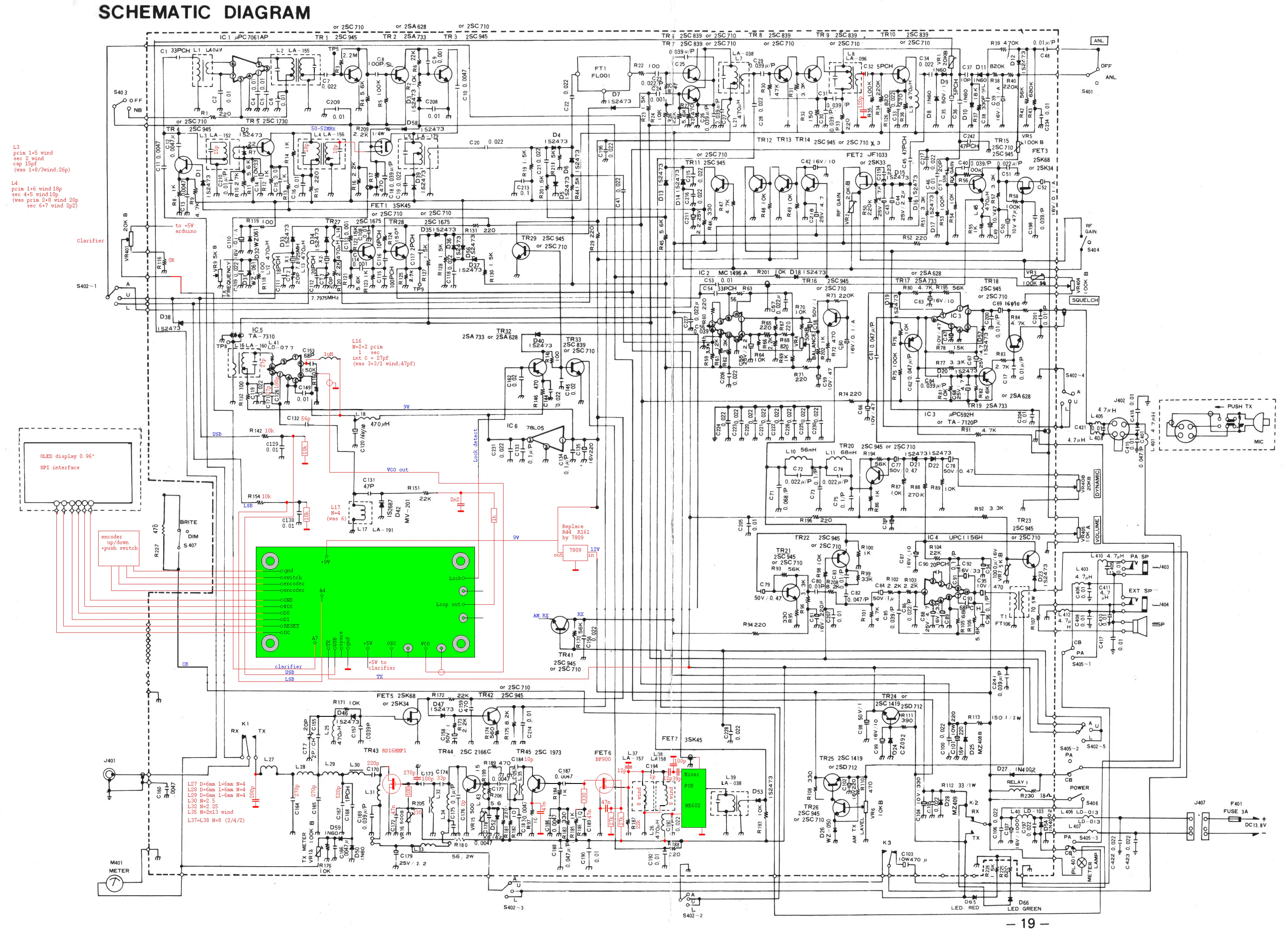Mixer 7.8 to 50...52MHz

This small PCB with a balanced mixer is used to replace the mixer dual gate mosfet. The FET must be replaced because of the needed suppression of the VCO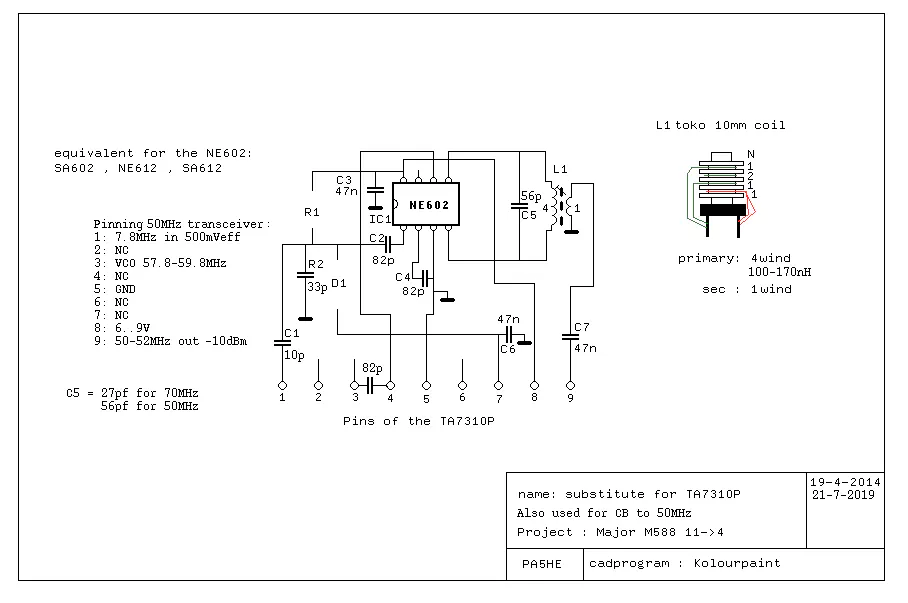Code

50MHz_OLED_PLL_DDS

Arduino
This software is used in an converted CB transceiver
It reads an encoder switch and sends data to an OLED display , PLL and DDS
/* Display for CB transceiver Arduino mini and SSD1606 Display
* Pins used :
* Display D0=4  D1=5 Reset =6  DC=7
* Encoder  pin 2 , pin3 , Switch =A0
* PLL      10 , 11 , 12
*/
#include <Arduino.h>
#include <U8g2lib.h>

#ifdef U8X8_HAVE_HW_SPI
#include <SPI.h>
#endif
#ifdef U8X8_HAVE_HW_I2C
#include <Wire.h>
#endif
#define pulseLow(pin) {digitalWrite(pin, LOW); digitalWrite(pin, HIGH); }

int encoderPin1 = 3;             // encoder
int encoderPin2 = 2;             // encoder
int lsbpin = 18  ;                   // assign LSB to pin D18/A4 pin D13 (spare) happen to be connected to a LED on the pro mini PCB
int usbpin = 8  ;                   // assign usb to D8
int txpin= 9;                         // assign tx to D9
int PLL_enb = 11;                 // assign PLL enable to D11
int PLL_dta = 10;                  // assign PLL data to D10
int PLL_clk = 12;                   // assign PLL clock to D12
int DDS_SDA = 17;
int DDS_SCL = 16;
int DDS_Fsync = 15;
volatile int lastEncoded = 0;    // encoder
long lastencoderValue = 0;       // encoder
int lastMSB = 0;                     // encoder
int lastLSB = 0;                      // encoder
int encoderValue = 0;            // encoder
long rx=100000;                     // Starting frequency of VFO in 1Hz steps
const char* hertz = "PA5HE";
int  hertzPosition = 5;
long rx2=1;                    // variable to hold the updated frequency
long increment = 10000;     // starting VFO update increment in 10kHZ.
int buttonstate = 0;                          // if the button is pressed or not
int memstatus = 1;                          // value to notify if memory is current or old. 0=old, 1=current.
int_fast32_t timepassed = millis(); // int to hold the arduino millis since startup
int mode, oldmode =0;
int oldpotmeterValue=512;   //mid position potmeter
/*
U8glib Example Overview:
Frame Buffer Examples: clearBuffer/sendBuffer. Fast, but may not work with all Arduino boards because of RAM consumption
Page Buffer Examples: firstPage/nextPage. Less RAM usage, should work with all Arduino boards.
U8x8 Text Only Example: No RAM usage, direct communication with display controller. No graphics, 8x8 Text only.

This is a page buffer example.
*/

// Please UNCOMMENT one of the contructor lines below
// U8g2 Contructor List (Picture Loop Page Buffer)
// The complete list is available here: https://github.com/olikraus/u8g2/wiki/u8g2setupcpp
// Please update the pin numbers according to your setup. Use U8X8_PIN_NONE if the reset pin is not connected
U8G2_SSD1306_128X64_NONAME_1_4W_SW_SPI u8g2(U8G2_R0, /* clock=*/ 4, /* data=*/ 5, /* cs=*/ U8X8_PIN_NONE, /* dc=*/ 7, /* reset=*/ 6);
//U8G2_SSD1306_128X64_NONAME_1_4W_HW_SPI u8g2(U8G2_R0, /* cs=*/ 12, /* dc=*/ 4, /* reset=*/ 6);	// Arduboy (Production, Kickstarter Edition)
//U8G2_SSD1306_128X64_NONAME_1_4W_HW_SPI u8g2(U8G2_R0, /* cs=*/ 10, /* dc=*/ 9, /* reset=*/ 8);
//U8G2_SSD1306_128X64_NONAME_1_3W_SW_SPI u8g2(U8G2_R0, /* clock=*/ 13, /* data=*/ 11, /* cs=*/ 10, /* reset=*/ 8);
//U8G2_SSD1306_128X64_NONAME_1_HW_I2C u8g2(U8G2_R0, /* reset=*/ U8X8_PIN_NONE);
//U8G2_SSD1306_128X64_NONAME_1_SW_I2C u8g2(U8G2_R0, /* clock=*/ 13, /* data=*/ 11, /* reset=*/ 8);
//U8G2_SSD1306_128X64_NONAME_1_SW_I2C u8g2(U8G2_R0, /* clock=*/ SCL, /* data=*/ SDA, /* reset=*/ U8X8_PIN_NONE);   // All Boards without Reset of the Display
//U8G2_SSD1306_128X64_NONAME_1_6800 u8g2(U8G2_R0, 13, 11, 2, 3, 4, 5, 6, A4, /*enable=*/ 7, /*cs=*/ 10, /*dc=*/ 9, /*reset=*/ 8);
//U8G2_SSD1306_128X64_NONAME_1_8080 u8g2(U8G2_R0, 13, 11, 2, 3, 4, 5, 6, A4, /*enable=*/ 7, /*cs=*/ 10, /*dc=*/ 9, /*reset=*/ 8);
//U8G2_SSD1306_128X64_VCOMH0_1_4W_HW_SPI u8g2(U8G2_R0, /* cs=*/ 10, /* dc=*/ 9, /* reset=*/ 8);	// same as the NONAME variant, but maximizes setContrast() range
// End of constructor list

void setup(void) {
u8g2.begin();
pinMode(encoderPin1, INPUT);
pinMode(encoderPin2, INPUT);
pinMode(usbpin,INPUT);
pinMode(lsbpin,INPUT);
pinMode(txpin,INPUT);
digitalWrite(encoderPin1, HIGH);           //turn pullup resistor on
digitalWrite(encoderPin2, HIGH);           //turn pullup resistor on
attachInterrupt(0, updateEncoder, CHANGE); //interrupt connected to the encoder
attachInterrupt(1, updateEncoder, CHANGE);
pinMode(A0,INPUT);                         // Connect to a button that goes to GND on push
digitalWrite(A0,HIGH);                     //turn pullup resistor on
pinMode(PLL_enb, OUTPUT);                  // All pins connected to the PLL are outputs
pinMode(PLL_dta, OUTPUT);
pinMode(PLL_clk, OUTPUT);
pinMode(DDS_Fsync, OUTPUT);
pinMode(DDS_SDA, OUTPUT);
pinMode(DDS_SCL, OUTPUT);
resetPLL();
setupDDS();
setupPLL();                 //set the PLL
//  Serial.begin(9600);  //for debugging
}

void loop(void) {
mode=lsb+usb+tx;
int frequencyerror = 400-512;                // add here the frequencyerror at 50MHz in Hz 512 is the offset from the clarifierpotmeter
int offset=0;                                            //has to do with the 7.8MHz IF of the Palomar
if ((rx != rx2) || (mode!=oldmode)||(potmeterValue!=oldpotmeterValue)){    //determine if something has changed
if (rx >=2000000){rx=rx2;};                                                                       // UPPER VFO LIMIT 52.000.0 MHz
if (rx <=0){rx=rx2;};                                                                                   // LOWER VFO LIMIT 50.000.0 MHz
if (tx){ offset=frequencyerror+2902-(lsb*5128);}
else {offset=frequencyerror+(usb*2390)-(lsb*2738)+potmeterValue;}                                  //tx switches the clarifier off
sendFrequency(rx+offset);                                                                               // frequency is send to the DDS
showFreq();                                                                                                       //frequency is send to the display
rx2 = rx;                                                                                                            //memory
if (rx2 >=2000000){rx2=2000000;};        // Correction in case there have been 2 interrupts
if (rx2 <=0){rx2=0;};                //
}
buttonstate = digitalRead(A0);                // button is connected to A0
oldmode=mode;
oldpotmeterValue=potmeterValue;
if(buttonstate == LOW) {
setincrement();
};
if(memstatus == 0){                    // Write the frequency to memory if not stored and 2 seconds have passed since the last frequency change.
if(timepassed+2000 < millis()){
//      storeMEM();
}
}
}

void setincrement(){
if(increment == 10){increment = 50; hertz = "50 Hz";}
else if (increment == 50){increment = 100;  hertz = "100 Hz";}
else if (increment == 100){increment = 500; hertz="500 Hz"; }
else if (increment == 500){increment = 1000; hertz="1 kHz"; }
else if (increment == 1000){increment = 5000; hertz="5 kHz"; }
else if (increment == 5000){increment = 10000; hertz="10 kHz"; }
else if (increment == 10000){increment = 100000; hertz="100 kHz";}
else{increment = 10; hertz = "10 Hz"; };
showFreq();
delay(200);   // added delay to get a more smooth reaction of the push button
}

void updateEncoder(){
int MSB = digitalRead(encoderPin1);        //MSB = most significant bit
int LSB = digitalRead(encoderPin2);         //LSB = least significant bit
int encoded = (MSB << 1) |LSB;               //converting the 2 pin value to single number
int sum = (lastEncoded << 2) | encoded;    //adding it to the previous encoded value
if(sum == 0b1101 || sum == 0b0100 || sum == 0b0010 || sum == 0b1011) encoderValue ++;
if(sum == 0b1110 || sum == 0b0111 || sum == 0b0001 || sum == 0b1000) encoderValue --;
if(encoderValue == 4) {rx=rx+increment ; encoderValue=0;} // there are 4 changes between one click of the encoder
if(encoderValue == -4) {rx=rx-increment ; encoderValue=0;}   // it can be neccessary to reduce these to 2 for other encoders
lastEncoded = encoded;                    //store this value for next time

}

void showFreq(){     //   0 frequency     1 frequencystep
char m_str;
byte millions=rx/1000000;             //MHz
byte hundredk = ((rx/100000)%10);     //100kHz
byte tenk = ((rx/10000)%10);               //10kHz
byte onek = ((rx/1000)%10);                //1kHz
byte hundreds = ((rx/100)%10);           //100Hz
byte tens =((rx/10)%10);                      //10Hz
u8g2.firstPage();
do {    u8g2.setFont(u8g2_font_logisoso16_tf);
u8g2.drawStr(0,39,"5");
u8g2.drawStr(10,39,strcpy(m_str, u8x8_u8toa(millions, 1)));
u8g2.drawStr(108,39,strcpy(m_str, u8x8_u8toa(hundreds, 1)));
u8g2.drawStr(118,39,strcpy(m_str, u8x8_u8toa(tens, 1)));
u8g2.drawStr(0,63,hertz);
u8g2.setFont(u8g2_font_logisoso38_tn);
u8g2.drawStr(18,39,".");
u8g2.drawStr(32,39,strcpy(m_str, u8x8_u8toa(hundredk, 1)));
u8g2.drawStr(56,39,strcpy(m_str, u8x8_u8toa(tenk, 1)));
u8g2.drawStr(80,39,strcpy(m_str, u8x8_u8toa(onek, 1)));
} while ( u8g2.nextPage() );
timepassed = millis();
memstatus = 0;           // Trigger memory write
};

void resetPLL(void){                          //RA2 enb RA0 clk RA1 dta
int counter=0;                           // enable high 5 clocks
digitalWrite(PLL_enb, HIGH);
digitalWrite(PLL_clk,LOW);
while (counter!=5 )
{  digitalWrite(PLL_clk, HIGH);
delayMicroseconds(3);
digitalWrite(PLL_clk, LOW);
delayMicroseconds(3) ;
++counter;   }
counter =0;
digitalWrite(PLL_clk, LOW);
digitalWrite(PLL_dta, LOW);
digitalWrite(PLL_enb, LOW);
delayMicroseconds(3);
while (counter!=3 )
{  digitalWrite(PLL_clk, HIGH);
delayMicroseconds(3);
digitalWrite(PLL_clk, LOW);
delayMicroseconds(3) ;
++counter;
}
digitalWrite(PLL_dta, HIGH);
delayMicroseconds(3);
digitalWrite(PLL_clk, HIGH);
delayMicroseconds(3);
digitalWrite(PLL_clk, LOW);
digitalWrite(PLL_dta, LOW);
delayMicroseconds(3);
digitalWrite(PLL_clk, HIGH);
delayMicroseconds(3);
digitalWrite(PLL_clk, LOW);
delayMicroseconds(3);
digitalWrite(PLL_enb, HIGH);
}

void sendFrequency(double frequency) {                            // frequency in hz above 50MHz
frequency +=  57800000 ;                                        //add IF and basefrequency
long freq = frequency *268435456/1470000000;    // 25 MHz clock on AD9833
// 1470 = 58.8 x 25Mhz
//       Serial.print(freq,DEC); // DEBUG
//       Serial.print("\n");
digitalWrite(DDS_Fsync, LOW);
tfr_byte(0x2000);                     //control word

digitalWrite(DDS_Fsync, HIGH);
digitalWrite(DDS_Fsync, LOW);

tfr_byte(0x4000|(freq & 0x3FFF)); //LSB first
freq>>=14;
tfr_byte(0x4000|(freq & 0x3FFF));  //MSB next
digitalWrite(DDS_Fsync, HIGH);
}

void tfr_byte(unsigned int data)  {                       // transfers 16 bits, MSB first to the 9833 via serial DDS_SDA line
for (int i=0; i<16; i++, data<<=1) {
if ((data&0x8000) == 0) { digitalWrite(DDS_SDA,0);}
else                               {digitalWrite(DDS_SDA,1); }
pulseLow(DDS_SCL);                                         //after each bit sent, CLK is pulsed low
}

}

void pllregister(unsigned int count,unsigned int bits){
digitalWrite(PLL_enb, LOW);
digitalWrite(PLL_clk, LOW);
while (count!=0)
digitalWrite(PLL_dta, HIGH);              // dta to 1
delayMicroseconds(3);                       // not too fast
digitalWrite(PLL_clk, HIGH);              // clk to 1

}
else { digitalWrite(PLL_dta, LOW);          // dta to 0
delayMicroseconds(3);
digitalWrite(PLL_clk, HIGH);             // clk to 1

}
delayMicroseconds(3);
digitalWrite(PLL_clk, LOW);              // clk back to low
delayMicroseconds(3);
count--;
}
digitalWrite(PLL_enb, HIGH);
digitalWrite(PLL_clk, LOW);
}

void setupDDS(){
digitalWrite(DDS_Fsync, LOW);
tfr_byte(0x100);      // reset DDS
tfr_byte(0x2000);    // set DDS 0x2020=block 0x2000=sine
digitalWrite(DDS_Fsync, HIGH);
}

void setupPLL() {
pllregister (8,0x70) ;                           //write C register 70hex
pllregister (16,0x24C) ;                         //write N register N=588
pllregister (15,10);                             //write R register 10 = 100kHz
}

Credits

hvde

0 projects • 9 followers